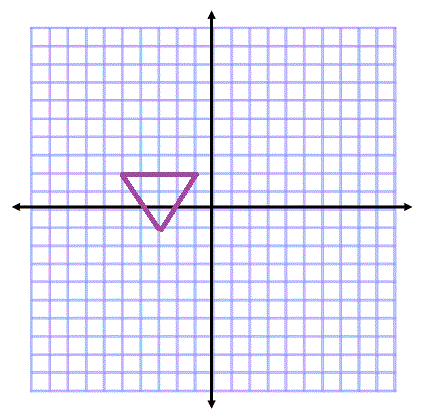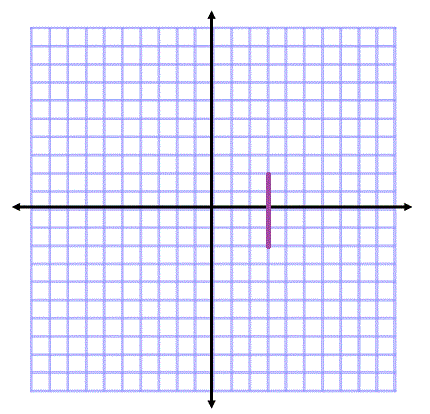# How to Graph Reflections Across Axes, the Origin, and Line y=x

Instructions:

Choose an answer and hit 'next'. You will receive your score and answers at the end.

question 1 of 3

### Which of the following is the rule for reflections across the x-axis?

Create Your Account To Take This Quiz

As a member, you'll also get unlimited access to over 75,000 lessons in math, English, science, history, and more. Plus, get practice tests, quizzes, and personalized coaching to help you succeed.

Try it risk-free for 30 days. Cancel anytime.

### 1. Which is the correct reflection of this figure across the line y = x?### 2. Which of the following shows the reflection of this line about the origin?Create your account to access this entire worksheet
A Premium account gives you access to all lesson, practice exams, quizzes & worksheets
Quizzes, practice exams & worksheets
Certificate of Completion
Create an account to get started

These practice materials cover the three types of reflections, a type of graphic transformation, and this quiz and worksheet combo will help you test your understanding of these transformations now and later. The quiz questions will ask you to identify types of reflections given sample graphs or sets of coordinates.

## Quiz & Worksheet Goals

In these assessments, you'll be tested on:

• The rules that govern reflections across both the x and y axes individually
• Identifying y=x reflections
• Identifying reflections around the point of origin
• Matching sets of coordinates to the coordinates that represent a certain type of reflection

## Skills Practiced

This quiz and worksheet will allow you to test your skills in the following areas:

• Reading comprehension - ensure that you draw the most important information on reflections from the related lesson
• Critical thinking - apply relevant concepts to examine information about transformations, particularly reflections, in a different light
• Problem solving - use your acquired knowledge to solve practice problems involving all three types of reflections
• Defining key concepts - ensure that you can accurately define key ideas, such as the three types of reflections - across the y or x axes, around the origin, or across the y=x line

There is also a related lesson called Graphing the Origin, Y=X & Reflections Across Axes. This lesson covers the following objectives:

• Define the three types of reflections
• Differentiate between the three types
• Define the rules for reflecting across the y and x axes individually
• Define the rule for reflecting across the origin
• Define the rule for reflecting across the y=x line
• Understand how to plug existing coordinates into these rules to obtain the reflection's points
Final ExamMath 103: Precalculus
Status: Not Started
Chapter ExamGraphing and Rational Equations
Status: Not Started

Support